# 【超详细】一文学会链表解题

danielmou

0收藏

### 前言

1. 什么是链表，链表的优缺点
2. 链表的表示及基本操作
3. 链表常见解题思路---翻转
4. 链表常见解题思路---快慢指针

### 什么是链表

#### 链表的定义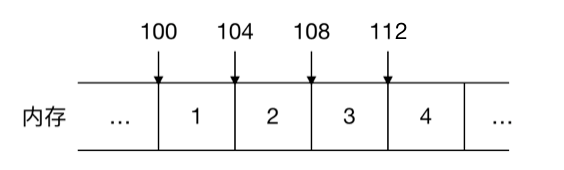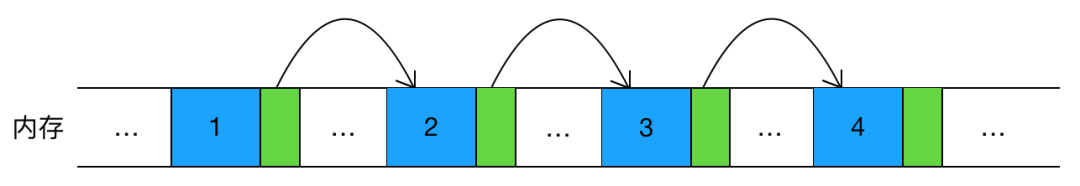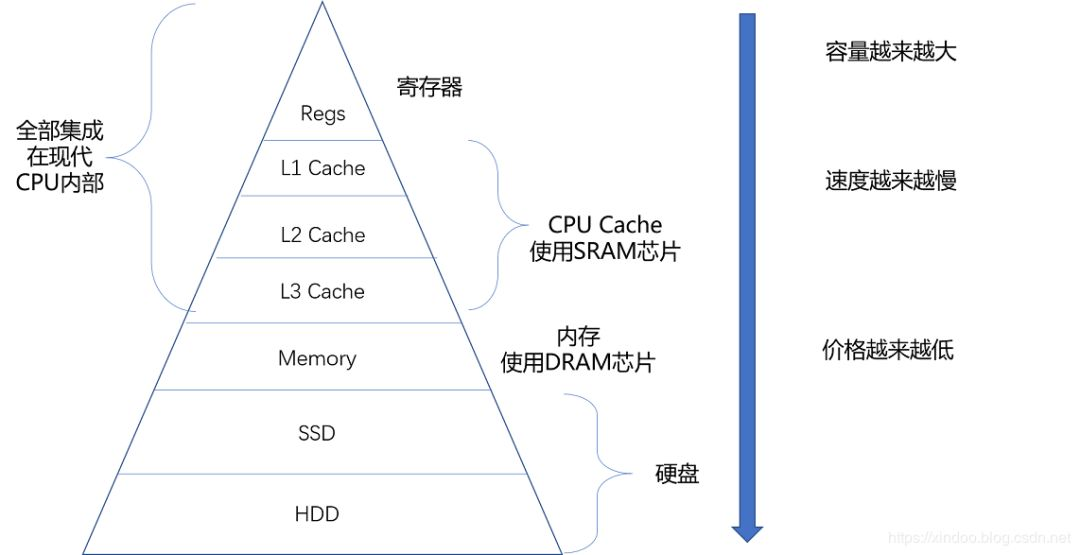• 大内存空间分配

• 元素频繁删除和插入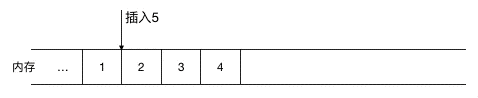(在 1，2 间插入 5，需要把2，3，4 同时往后移一位)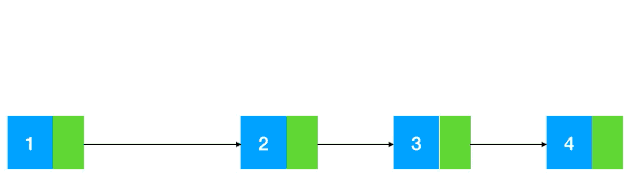### 链表的表示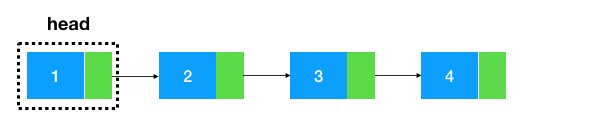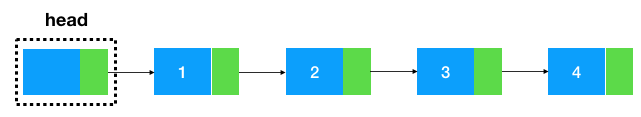``````/**
* 链表中的结点，data代表节点的值，next是指向下一个节点的引用
*/
class Node {
int data;// 结点的数组域，值
Node next =     null;// 节点的引用，指向下一个节点
public Node(    int{
this.data = data;
}
}

/**
* 链表
*/
int length = 0; // 链表长度，非必须，可不加
Node head =     null; // 头结点

}     else {
while (tmp.next !=     null) {
tmp = tmp.next;
}
tmp.next =     new Node(val);
}
}
}``````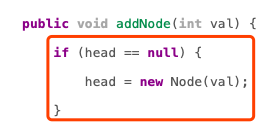1. 每插入一个元素都要对头结点进行判空比较，如果一个链表有很多元素需要插入，就需要进行很多次的判空处理，不是那么高效
2. 头结点与其他结点插入逻辑不统一（一个需要判空后再插入，一个不需要判空直接插入）,从程序逻辑性来说不是那么合理（因为结点与结点是平级，添加逻辑理应相同）

``````    public    class LinkedList {
int length = 0; // 链表长度，非必须，可不加
Node head =     new Node(0); // 哨兵结点
while (tmp.next !=     null) {
tmp = tmp.next;
}
tmp.next =     new Node(val);
length++
}
}``````

### 链表常见解题套路--翻转

#### 热身赛``````    public    class LinkedList {
int length = 0; // 链表长度，非必须，可不加
Node head =     new Node(0); // 哨兵节点

// 头插法
// 1.构造新结点
Node newNode =     new Node(val);
// 2.新结点指向头结点之后的结点
// 3.头结点指向新结点
length++
}

public     static     void main(String[] args){
int[] arr = {1,2,3,4};
// 头插法构造链表
for (    int i = 0; i < arr.length; i++) {
}
// 打印链表,将打印 4-->3-->2-->1
}
}``````

#### 小试牛刀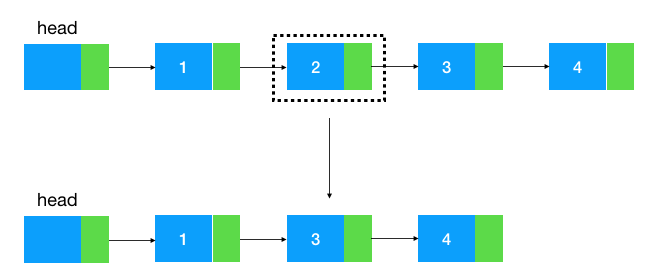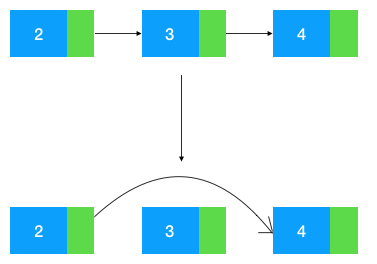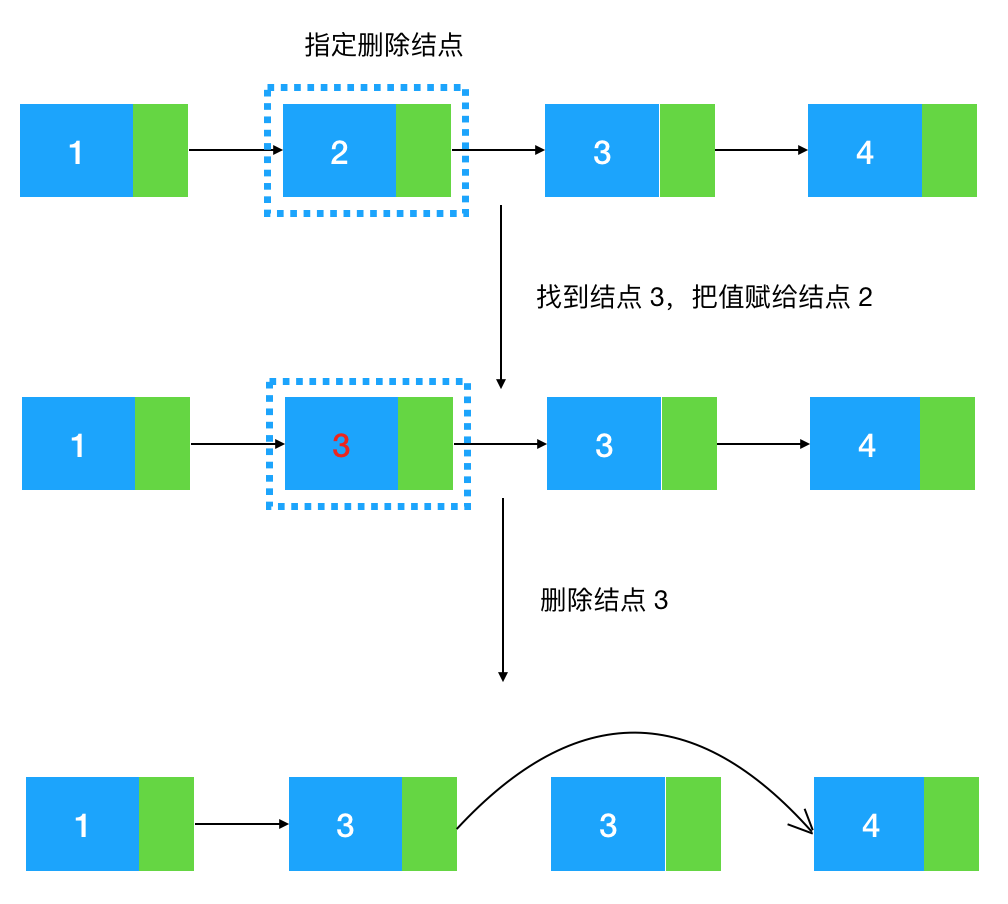``````/**
* 删除指定的结点
* @param deletedNode
*/
public     void removeSelectedNode(Node deletedNode){
// 如果此结点是尾结点我们还是要从头遍历到尾结点的前继结点，再将尾结点删除
if (deletedNode.next ==     null) {
while (tmp.next != deletedNode) {
tmp = tmp.next;
}
// 找到尾结点的前继结点，把尾结点删除
tmp.next =     null;
}     else {
Node nextNode = deletedNode.next;
// 将删除结点的后继结点的值赋给被删除结点
deletedNode.data = nextNode.data;
// 将 nextNode 结点删除
deletedNode.next = nextNode.next;
nextNode.next =     null;
}
}``````

#### 入门到进阶:链表翻转

• 递归翻转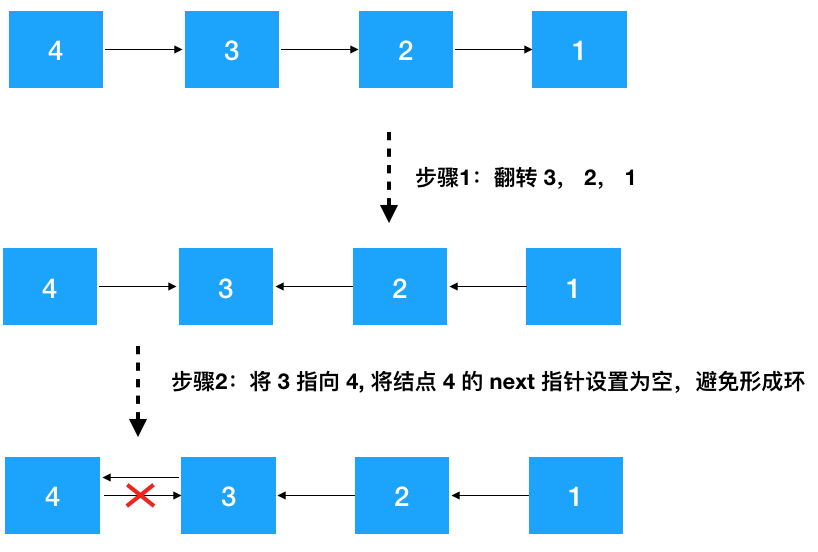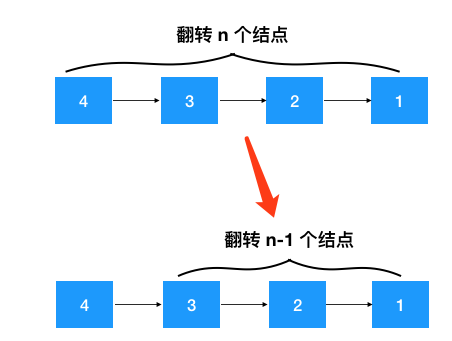1、定义递归函数,明确函数的功能 根据以上分析，这个递归函数的功能显然是翻转某个节点开始的链表，然后返回新的头结点

``````/**
* 翻转结点 node 开始的链表
*/
}``````

2、寻找递推公式 上文中已经详细画出了翻转链表的步骤，简单总结一下递推步骤如下

• 针对结点 node (值为 4), 先翻转 node 之后的结点   invert(node->next) ,翻转之后 4--->3--->2--->1变成了 4--->3<---2<---1
• 再把 node 节点的下个节点（3）指向 node，node 的后继节点设置为空（避免形成环），此时变成了 4<---3<---2<---1
• 返回新的头结点，因为此时新的头节点从原来的 4 变成了 1，需要重新设置一下 head

``````/**
* 递归翻转结点 node 开始的链表
*/
if (node.next ==     null) {
return node;
}

// 步骤 1: 先翻转 node 之后的链表

// 步骤 2: 再把原 node 节点后继结点的后继结点指向 node (4)，node 的后继节点设置为空(防止形成环)
node.next.next = node;
node.next =     null;

// 步骤 3: 返回翻转后的头结点
}

public     static     void main(String[] args){
int[] arr = {4,3,2,1};
for (    int i = 0; i < arr.length; i++) {
}
// 翻转后别忘了设置头结点的后继结点！
}``````

4、计算时间/空间复杂度 由于递归调用了 n 次 invertLinkedList 函数，所以时间复杂度显然是 O(n), 空间复杂度呢，没有用到额外的空间，但是由于递归调用了  n 次 invertLinkedList 函数，压了 n 次栈，所以空间复杂度也是 O(n)。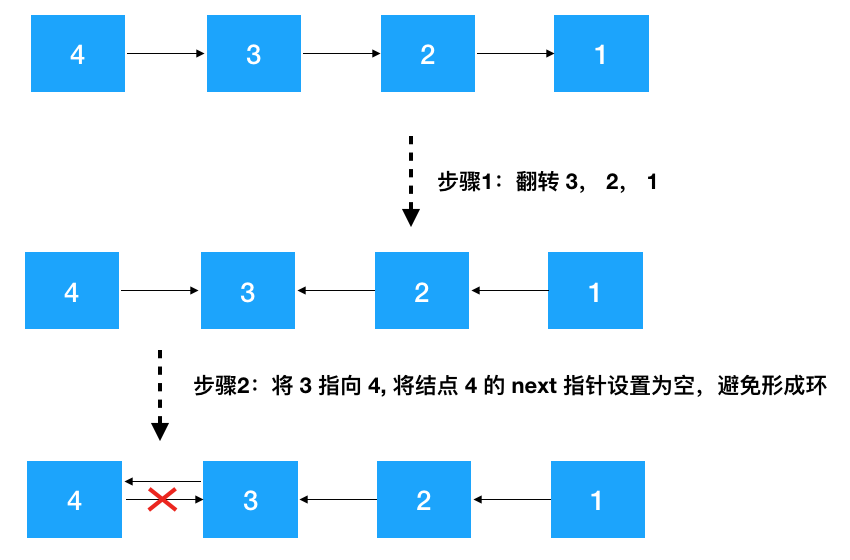• 非递归翻转链表（迭代解法）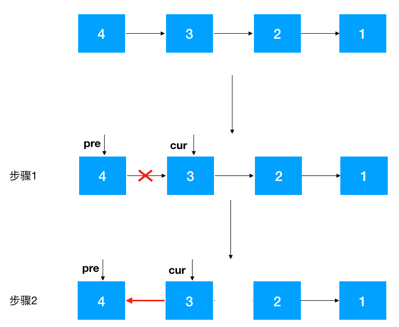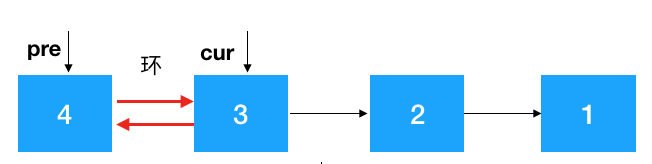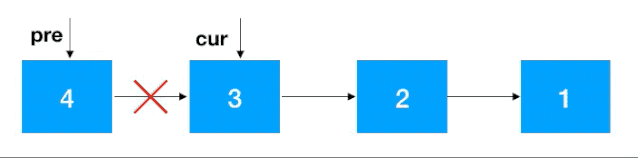``````/**
* 迭代翻转
*/
// 步骤 1
Node cur = pre.next;
pre.next =     null;

while (cur !=     null) {
/**
* 务必注意：在 cur 指向 pre 之前一定要先保留 cur 的后继结点，不然 cur 指向 pre 后就再也找不到后继结点了
* 也就无法对 cur 后继之后的结点进行翻转了
*/
Node next = cur.next;
cur.next = pre;
pre = cur;
cur = next;
}
// 此时 pre 为头结点的后继结点
}``````

1. 根据 from 和 to 找到 from-1, from, to,  to+1 四个结点(注意临界条件，如果 from 从头结点开始，则 from-1 结点为空, 翻转后需要把 to 设置为头结点的后继结点， from 和 to 结点也可能超过尾结点,这两种情况不符合条件不翻转)。
2. 对 from 到 to 的结点进行翻转
3. 将 from-1 节点指向 to 结点，将 from 结点指向 to + 1 结点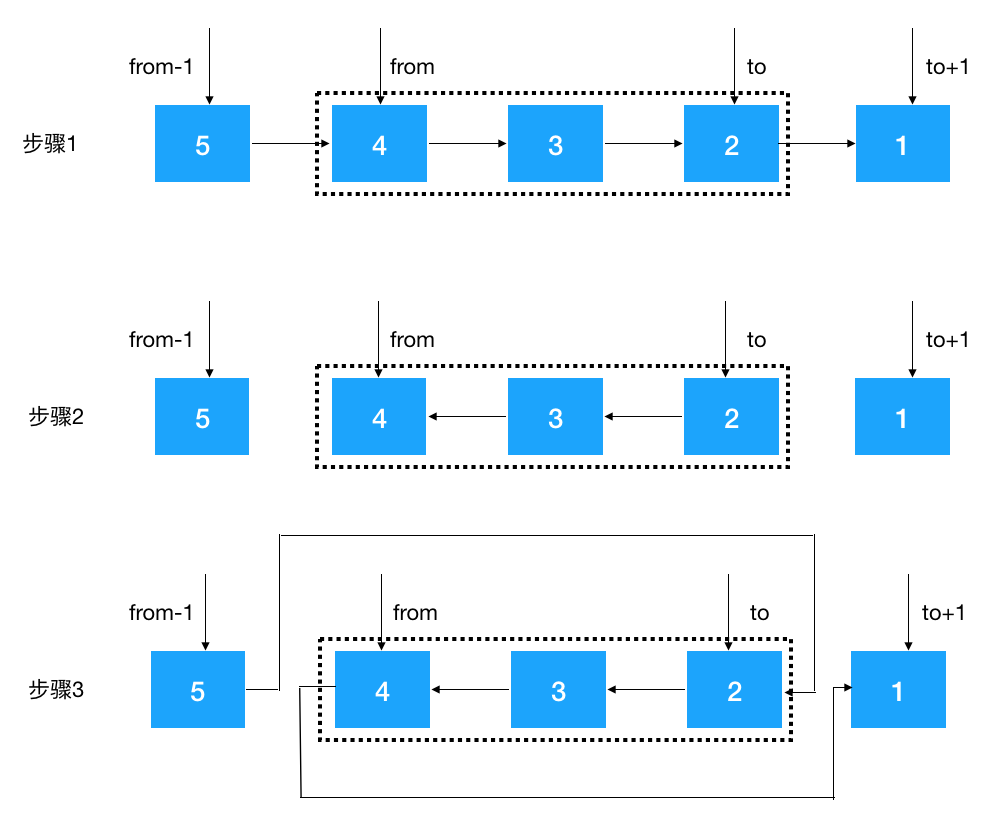``````/**
* 迭代翻转 from 到 to 的结点
*/
public     void iterationInvertLinkedList(    int fromIndex,     int     throws{

Node fromPre =     null;            // from-1结点
Node from =     null;               // from 结点
Node to =     null;                 // to 结点
Node toNext =     null;             // to+1 结点

// 步骤 1：找到  from-1, from, to,  to+1 这四个结点
int curIndex = 1;      // 头结点的index为1
while (tmp !=     null) {
if (curIndex == fromIndex-1) {
fromPre = tmp;
}     else    if (curIndex == fromIndex) {
from = tmp;
}     else    if (curIndex == toIndex) {
to = tmp;
}     else    if (curIndex == toIndex+1) {
toNext = tmp;
}
tmp = tmp.next;
curIndex++;
}

if (from ==     null || to ==     null) {
// from 或 to 都超过尾结点不翻转
throw    new Exception("不符合条件");
}

// 步骤2：以下使用循环迭代法翻转从 from 到 to 的结点
Node pre = from;
Node cur = pre.next;
while (cur != toNext) {
Node next = cur.next;
cur.next = pre;
pre = cur;
cur = next;
}

// 步骤3：将 from-1 节点指向 to 结点（如果从 head 的后继结点开始翻转，则需要重新设置 head 的后继结点），将 from 结点指向 to + 1 结点
if (fromPre !=     null) {
fromPre.next = to;
}     else {
}
from.next = toNext;
}``````

• 你的算法只能使用常数的额外空间。
• 你不能只是单纯的改变节点内部的值，而是需要实际的进行节点交换。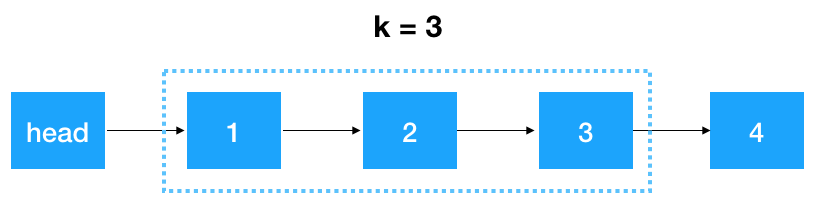• 首先，我们要记录 3 个一组这一段链表的前继结点,定义为 startKPre,然后再定义一个 step, 从这一段的头结点 (1）开始遍历 2 次，找出这段链表的起始和终止结点，如下图示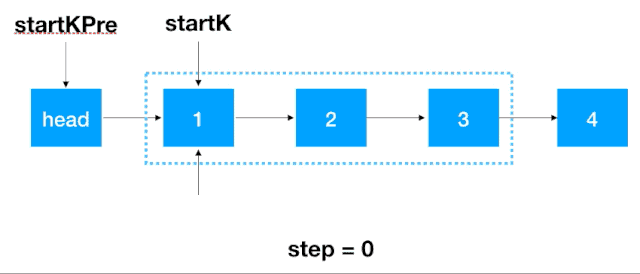• 找到 startK 和 endK 之后，根据之前的迭代翻转法对 startK 和 endK 的这段链表进行翻转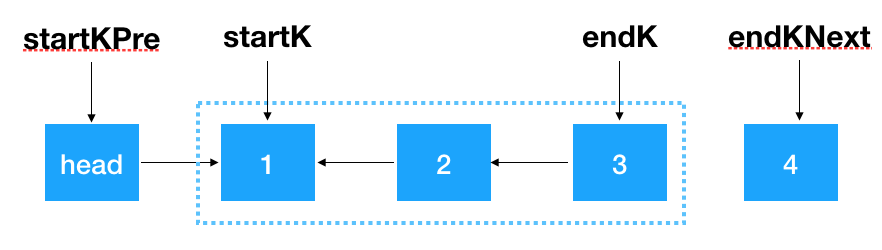• 然后将 startKPre 指向 endK,将 startK 指向 endKNext，即完成了对 k 个一组结点的翻转。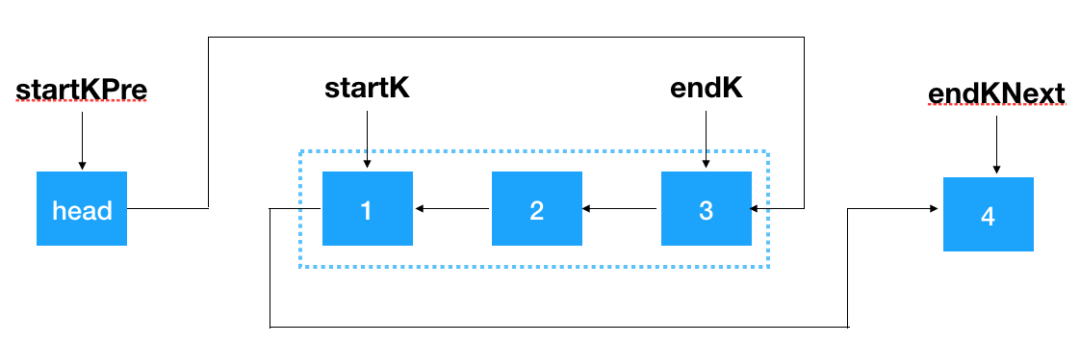``````/**
* 每 k 个一组翻转链表
* @param k
*/
int step = 0;               // 计数，用来找出首结点和尾结点

Node startK =     null;         // k个一组链表中的头结点
Node startKPre = head;      // k个一组链表头结点的前置结点
Node endK;                  // k个一组链表中的尾结点
while (tmp !=     null) {
// tmp 的下一个节点,因为由于翻转，tmp 的后继结点会变,要提前保存
Node tmpNext = tmp.next;
if (step == 0) {
// k 个一组链表区间的头结点
startK = tmp;
step++;
}     else    if (step == k-1) {
// 此时找到了 k 个一组链表区间的尾结点（endK）,对这段链表用迭代进行翻转
endK = tmp;
Node pre = startK;
Node cur = startK.next;
if (cur ==     null) {
break;
}
Node endKNext = endK.next;
while (cur != endKNext) {
Node next = cur.next;
cur.next = pre;
pre = cur;
cur = next;
}
// 翻转后此时 endK 和 startK 分别是是 k 个一组链表中的首尾结点
startKPre.next = endK;
startK.next = endKNext;

// 当前的 k 个一组翻转完了，开始下一个 k 个一组的翻转
startKPre = startK;
step = 0;
}     else {
step++;
}
tmp = tmpNext;
}
}``````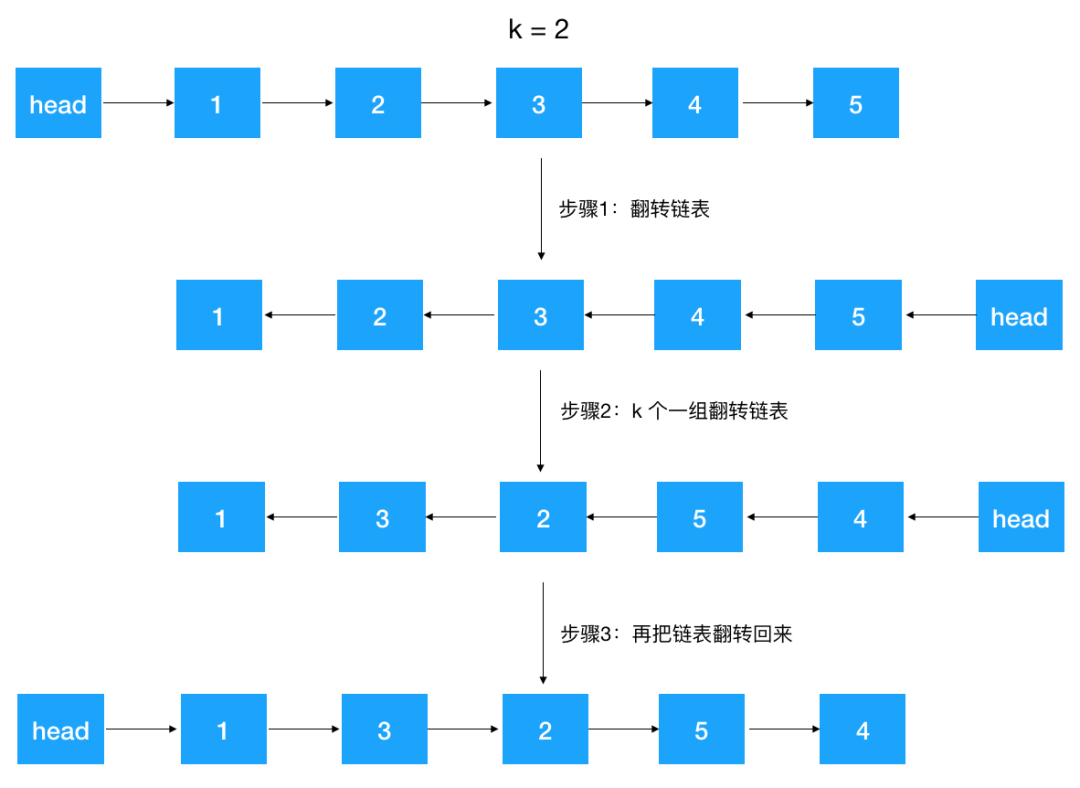``````/**
* 逆序每 k 个一组翻转链表
* @param k
*/
// 先翻转链表
// k 个一组翻转链表
// 再次翻转链表
}``````

### 链表解题利器---快慢指针

1. 寻找/删除第 K 个结点
2. 有关链表环问题的相关解法

#### 寻找/删除第 K 个结点

##### 小试牛刀之一

1. 假如链表长度是奇数: head--->1--->2--->3--->4--->5, 从 1 开始遍历 5/2 = 2 （取整）次，到达 3，3确实是中间结点
2. 假如链表长度是偶数: head--->1--->2--->3--->4--->5--->6, 从 1 开始遍历 6/2 = 3次，到达 4，4 确实是中间结点的第二个结点

``````    public Node findMiddleNode(){
int middleLength = length / 2;
while (middleLength > 0) {
tmp = tmp.next;
middleLength--;
}
return tmp;
}``````

``````    public Node findMiddleNodeWithoutHead(){
int length = 1;
// 选遍历一遍拿到链表长度
while (tmp.next !=     null) {
tmp = tmp.next;
length++;
}

// 再遍历一遍拿到链表中间结点
int middleLength = length / 2;
while (middleLength > 0) {
tmp = tmp.next;
middleLength--;
}
return tmp;
}``````

• 如果链表长度为奇数,当 fast.next = null 时,slow 为中间结点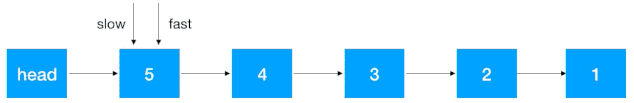• 如果链表长度为偶数,当 fast = null 时,slow 为中间结点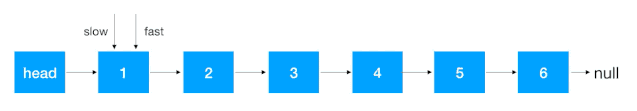``````/**
* 使用快慢指针查找找到中间结点
* @return
*/
public Node findMiddleNodeWithSlowFastPointer(){
while (fast !=     null && fast.next !=     null) {
// 快指针走两步
fast = fast.next.next;
// 慢指针走一步
slow = slow.next;
}
// 此时的 slow 结点即为哨兵结点
return slow;
}``````

2. 快指针往前走 k- 1 步,先走到第 k 个结点
3. 快慢指针同时往后走一步，不断重复此步骤，直到快指针走到尾结点,此时的 slow 结点即为我们要找的倒序第 k 个结点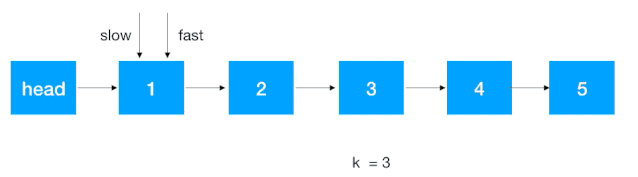``````    public Node findKthToTail(    int     throws{

// 快指针先移到第k个结点
int tmpK = k - 1;
while (tmpK > 0 && fast !=     null) {
fast = fast.next;
tmpK--;
}
// 临界条件：k大于链表长度
if (fast ==     null) {
throw    new Exception("K结点不存在异常");
}
// slow 和 fast 同时往后移，直到 fast 走到尾结点
while (fast.next !=     null) {
slow = slow.next;
fast = fast.next;
}
return slow;
}``````

• 先找到倒数第 K+1 个结点, 此结点的后继结点即为倒数第 K 个结点
• 将倒数第 K+1 结点的的后继结点设置为 null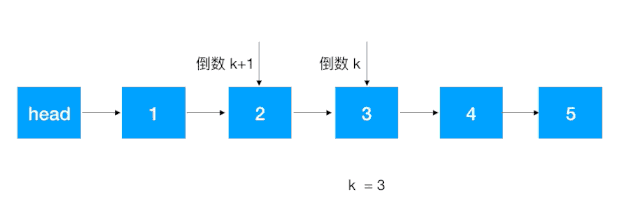``````    public     void reversedKthToTail(    int     throws{
// 直接调已实现的 寻找倒序k个结点的方法，这里是 k+1
Node KPreNode = findKthToTail(k+1);
// 倒数第 K 个结点
Node kNode = KPreNode.next;

KPreNode.next =     null;

// 寻找尾结点
Node tmp = kNode;
while (tmp.next !=     null) {
tmp = tmp.next;
}
}``````

##### 小试牛刀之二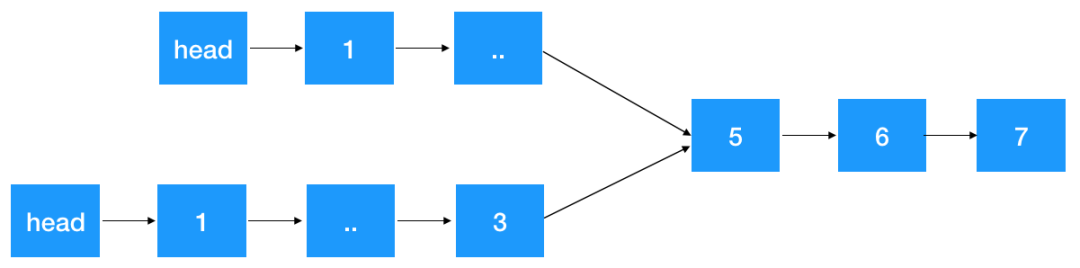1、如果链表没有定义长度，则我们先遍历这两个链表拿到两个链表长度，假设分别为 L1, L2 (L1 >= L2), 定义 p1, p2 指针分别指向各自链表 head 结点，然后 p1 先往前走 L1 - L2 步。这一步保证了 p1，p2 指向的指针与相交结点(如果有的话)一样近。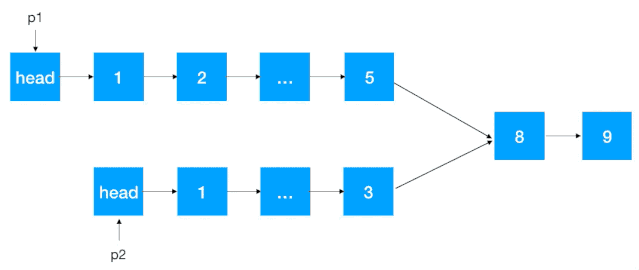2、 然后 p1,p2 不断往后遍历，每次走一步，边遍历边判断相应结点是否相等，如果相等即为这两个链表的相交结点

``````    public     static Node detectCommonNode(LinkedList list1, LinkedList list2){
int length1 = 0;        // 链表 list1 的长度
int length2 = 0;        // 链表 list2 的长度

while (p1.next !=     null) {
length1++;
p1 = p1.next;
}

while (p2.next !=     null) {
length2++;
p2 = p2.next;
}

// p1 或 p2 前进 |length1-length2| 步
if (length1 >= length2) {
int diffLength = length1-length2;
while (diffLength > 0) {
p1 = p1.next;
diffLength--;
}
}     else {
int diffLength = length2-length1;
while (diffLength > 0) {
p2 = p2.next;
diffLength--;
}
}
// p1,p2分别往后遍历，边遍历边比较，如果相等，即为第一个相交结点
while (p1 !=     null && p2.next !=     null) {
p1 = p1.next;
p2 = p2.next;
if (p1.data == p2.data) {
// p1，p2 都为相交结点，返回 p1 或 p2
return p1;
}
}
// 没有相交结点，返回空指针
return    null;
}``````
##### 进阶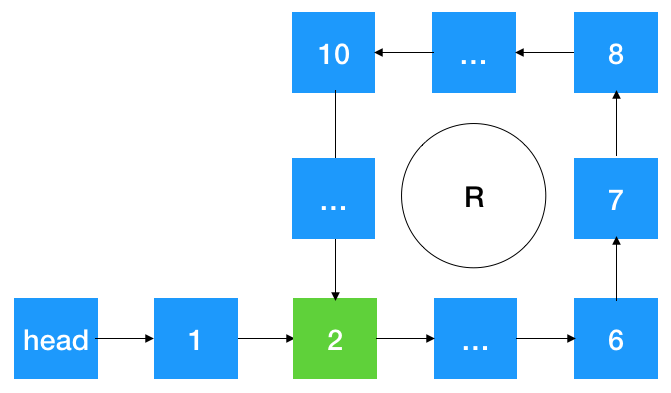1、 假如快指针离慢指针相差一个结点,则再一次遍历，慢指针走一步，快指针走两步，相遇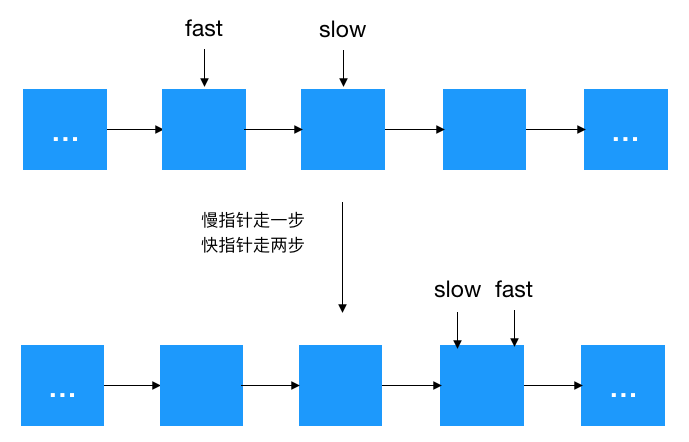2、  假如快指针离慢指针相差两个结点,则再一次遍历，慢指针走一步，快指针走两步，相差一个结点，转成上述 1 的情况

3、 假如快指针离慢指针相差 N 个结点（N大于2），则下一次遍历由于慢指针走一步，快指针走两步，所以相差 N+1-2 = N-1 个结点，发现了吗，相差的结点从 N 变成了 N-1,缩小了！不断地遍历，相差的结点会不断地缩小，当 N 缩小为 2 时，即转为上述步骤 2 的情况，由此得证，如果有环，快慢指针一定会相遇！

``````/**
* 判断是否有环,返回快慢指针相遇结点,否则返回空指针
*/
public Node detectCrossNode(){

while (fast !=     null && fast.next !=     null) {
fast = fast.next.next;
slow = slow.next;

if (fast ==     null) {
return    null;
}

if (slow == fast) {
return slow;
}
}
return     null;
}``````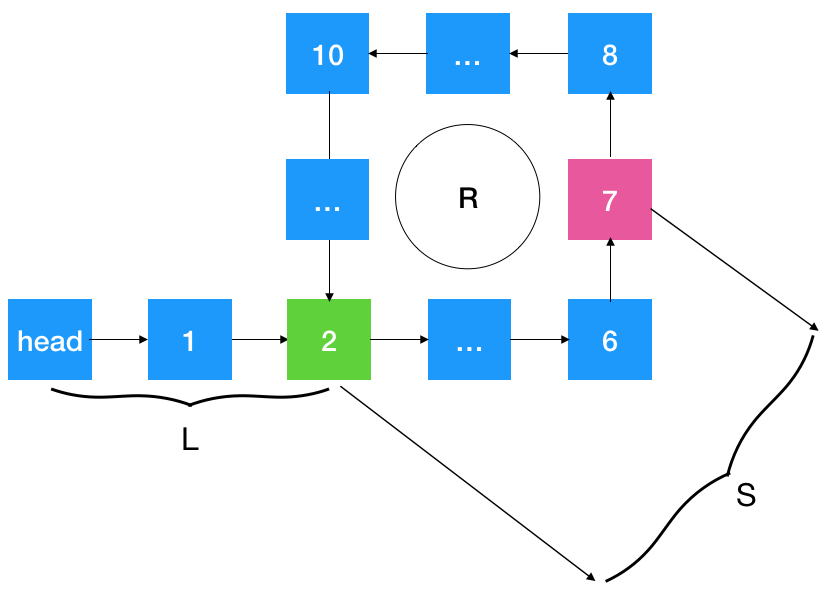• 当 n = 1 时，则 L+S = R 时,则从相遇点 7 开始遍历走到环入口点 2 的距离为 R - S = L，刚好是环的入口结点,而 head 与环入口点 2 的距离恰好也为 L，所以只要在头结点定义一个指针，在相遇点（7）定义另外一个指针，两个指针同时遍历，每次走一步，必然在环的入口位置 2 相遇
• 当 n > 1 时，L + S = nR,即 L = nR - S,  nR-S 怎么理解？可以看作是指针从结点  7 出发，走了 n 圈后，回退 S 步，此时刚好指向环入口位置，也就是说如果设置一个指针指向 head（定义为p1）, 另设一个指针指向 7（定义为p2），不断遍历，p2 走了 nR-S 时（即环的入口位置），p1也刚好走到这里（此时 p1 走了 nR-S =  L步，刚好是环入口位置），即两者相遇！

``````    public Node getRingEntryNode(){
// 获取快慢指针相遇结点
Node crossNode = detectCrossNode();

// 如果没有相遇点，则没有环
if (crossNode ==     null) {
return    null;
}

// 分别定义两个指针，一个指向头结点，一个指向相交结点
Node tmp2 = crossNode;

// 两者相遇点即为环的入口结点
while (tmp1.data != tmp2.data) {
tmp1 = tmp1.next;
tmp2 = tmp2.next;
}
return tmp1;
}``````

### 总结帖子
视频
声望
粉丝
社区精华内容